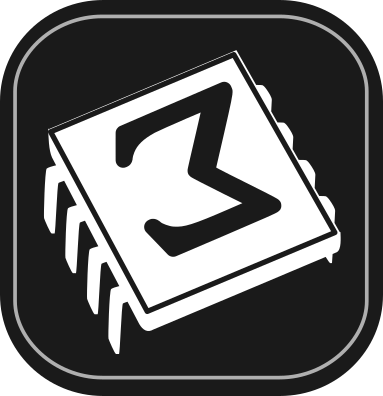[main]Notes on TeXmacs

# Example of TikZ figure embedding within a document

Besides generating TikZ figures within a Graph plugin session, it is possible to embed them directly in a document, so that they are displayed as an image (without the code and the plugin prompt), while keeping them editable.

See HelpManualUse TeXmacs as an interface and its Section 4: Plug-ins as scripting language for a general description of the facility.

Let us see here how to use the Fold environment to embed an editable TikZ picture in a TeXmacs document, making in this way TikZ a flexible graphing tool for TeXmacs.

The first step is inserting an executable field using the menu:

InsertFoldExecutableGraph

(there are more options under InsertFoldExecutable, but Graph is the most recent plugin with the most flexible functionality).

This results in a yellow-colored field, introduced by the “Graph” title on a gray background:

 Graph

Press ⇧Return to start typing or pasting code; pressing Return now would immediately compile the yet-empty code, yielding a blank figure. Once ⇧Return has been pressed for the first time and it becomes possible to type code, the effects of the keys change: ⇧Return itself executes the code (generates the image), while Return inserts a new line (the behaviour of the Return and ⇧Return key combinations is described in the Manual at HelpManualUse TeXmacs as an interface in its Section 3).

As a first example, let us choose one based on TikZ only, that does not need any further LaTeX packages, taking it from the guide “A very minimal introduction to TikZ” by Jacques Crémer (available at https://cremeronline.com/LaTeX/minimaltikz.pdf, see section 4.1). Since we want to use the TikZ option of the Graph plugin, the first line in our code has to consist of the characters %tikz :

 Graph %tikz \begin{tikzpicture} \draw [fill=red,ultra thick] (0,0) rectangle (1,1); \draw [fill=red,ultra thick,red] (2,0) rectangle (3,1); \draw [blue, fill=blue] (4,0) -- (5,1) -- (4.75,0.15) -- (4,0); \draw [fill] (7,0.5) circle [radius=0.1]; \draw [fill=orange] (9,0) rectangle (11,1); \draw [fill=white] (9.25,0.25) rectangle (10,1.5); \end{tikzpicture}

Press ⇧Return to execute the code and obtain after a few seconds the TikZ picture: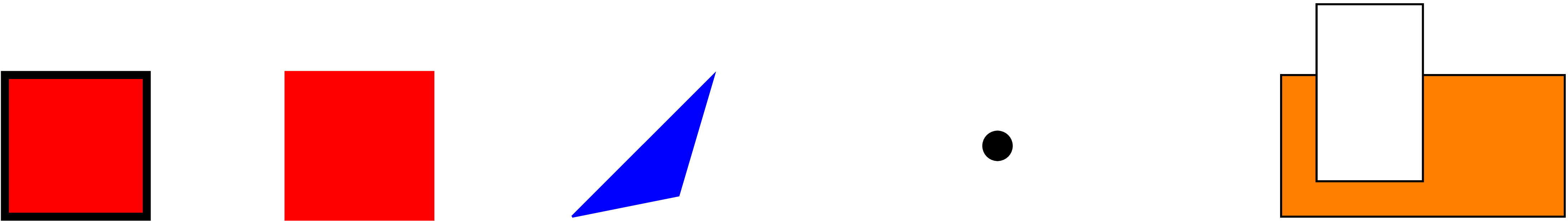The appearance within the TeXmacs editor is a bit rough, but it is fine in the converted pdf documents.

The executable Fold environment with the TikZ picture can be placed inside a TeXmacs image environment: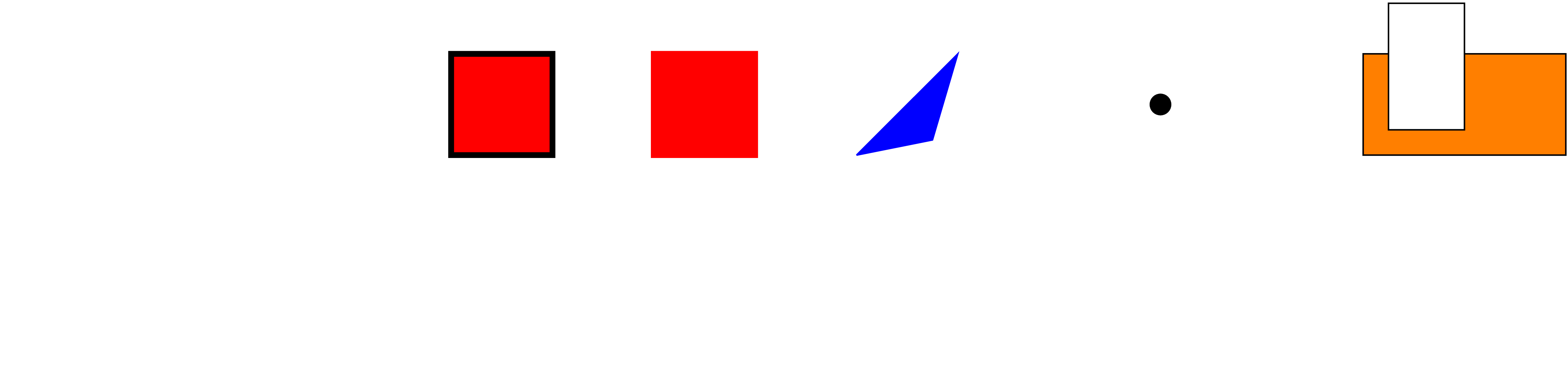Figure 1. TikZ example from Jacques Crémer's “A very minimal introduction to TikZ”

Placing the cursor at the generated figure (just after it: the cursor becomes as tall as the figure) makes two functions available.

First function: get back to the executable field

With the cursor at the figure (in this case, it can be placed before the figure as well as after it), press Return and obtain back the executable field, with the code ready for editing:

 Graph %tikz \begin{tikzpicture} \draw [fill=red,ultra thick] (0,0) rectangle (1,1); \draw [fill=red,ultra thick,red] (2,0) rectangle (3,1); \draw [blue, fill=blue] (4,0) -- (5,1) -- (4.75,0.15) -- (4,0); \draw [fill] (7,0.5) circle [radius=0.1]; \draw [fill=orange] (9,0) rectangle (11,1); \draw [fill=white] (9.25,0.25) rectangle (10,1.5); \end{tikzpicture}

Press ⇧Return to compile and display again. This process can be repeated as many times as one wishes.

Second function: get the inactivated image macro

Again with the cursor at the figure (in this case only after the figure: placing it before the figure does not make the function we are describing available), press and obtain the inactivated TeXmacs image macro:

<image||0.618par|||>

The script is now invisible (it is inside a hidden <script-output||||> macro).

One possibility here is to adjust the width of the image: as an example, place the cursor in the field with the width and change it from 0.618par to 0.9par

<image||0.9par|||>

press Return to get back to the figure, which has now become larger: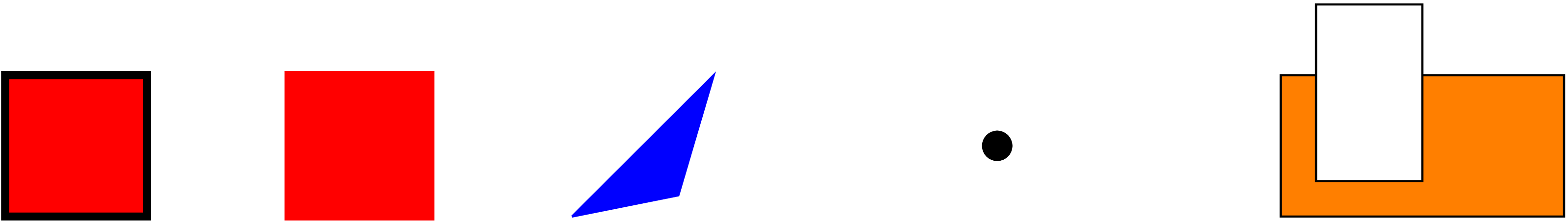The initial script is again accessible (with the Return key), but accessing and executing it resets the figure size to 0.618par.

The other parameter fields in the figure macro can be used to alter the height of the image (second parameter, in this case the image proportions are not preserved) and its placement with respect to the insertion point (third and fourth parameters):

<image||0.5par||0.2par|0.1par>

and again activating with Return obtain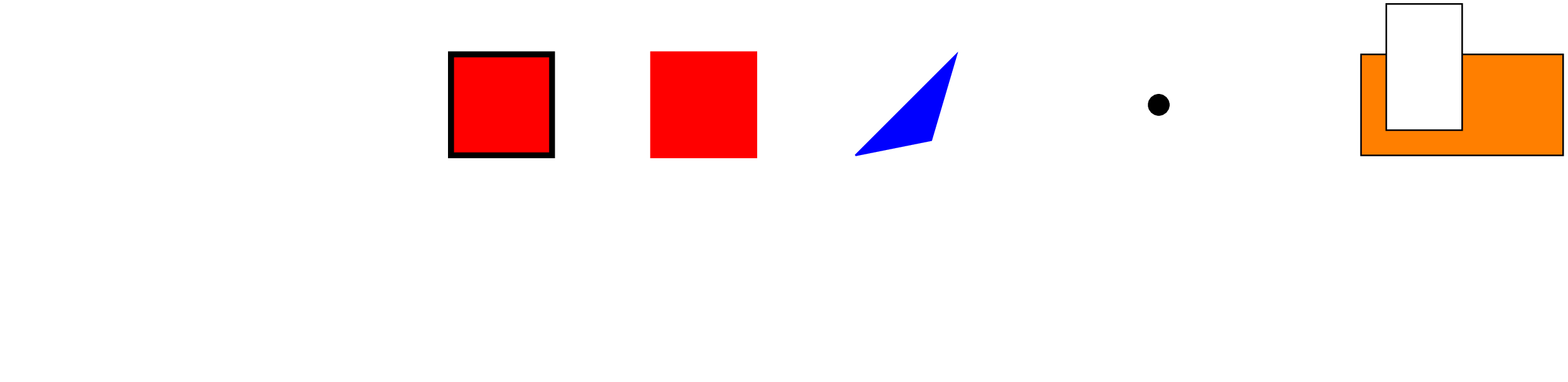The white space above the line you are reading now is part of the image field, where the image itself has been sized down and shifted right and up by the change of the macro parameters.

## More complex pictures

As in Graph plugin sessions, one can place in fold executables complex TikZ programs that depend on other LaTeX packages. In this case one should insert in the executable field the whole LaTeX document (the preferred class is standalone); the first line in this case must consist of the characters %pdflatex .

Here is the same code (copied from this TeX StackExchange question) as in Example of TikZ figure generation with the Graph plugin; it needs the package tikz-3dplot:

 Graph %pdflatex \documentclass[tikz]{standalone} \usepackage{tikz-3dplot} \begin{document} \tdplotsetmaincoords{60}{120} \begin{tikzpicture}[ scale=3, tdplot_main_coords, axis/.style={->,blue,thick}, vector/.style={-stealth,red,very thick}, vector guide/.style={dashed,red,thick}] %standard tikz coordinate definition using x, y, z coords \coordinate (O) at (0,0,0); %tikz-3dplot coordinate definition using r, theta, phi coords \tdplotsetcoord{P}{.8}{55}{60} %draw axes \draw[axis] (0,0,0) -- (1,0,0) node[anchor=north east]{$x$}; \draw[axis] (0,0,0) -- (0,1,0) node[anchor=north west]{$y$}; \draw[axis] (0,0,0) -- (0,0,1) node[anchor=south]{$z$}; %draw a vector from O to P \draw[vector] (O) -- (P); %draw guide lines to components \draw[vector guide] (O) -- (Pxy); \draw[vector guide] (Pxy) -- (P); \end{tikzpicture} \end{document}

Executing the code (press ⇧Return) yields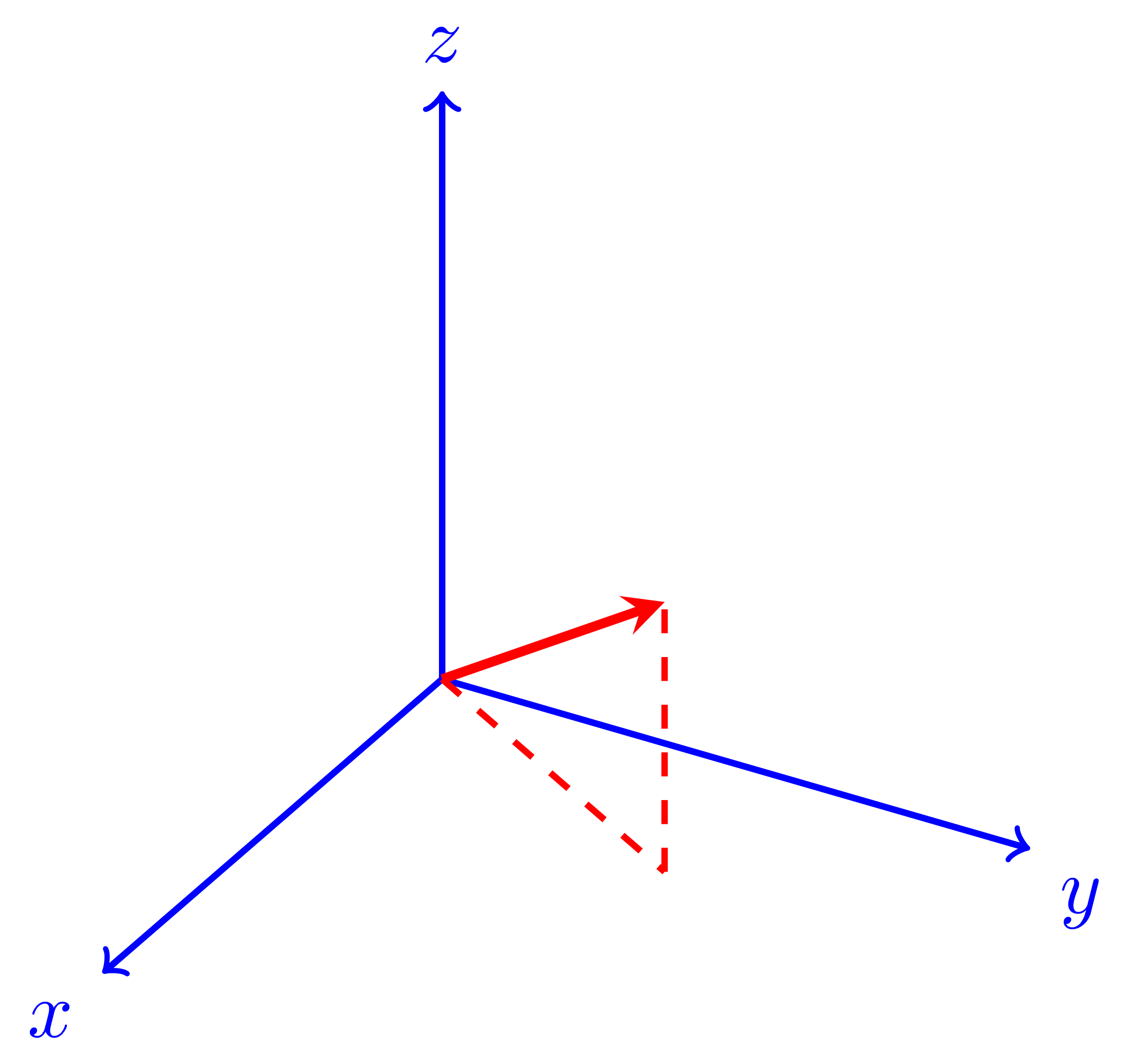Once more, the Fold environment with the LaTeX code can be placed anywhere in a TeXmacs document, making it possible for example to place complex TikZ pictures inside TeXmacs image environments seamlessly.

## Error messages and troubleshooting

• If the first line does not correspond to any of the backends known to Graph, compilation will display a notice (if for example the first line is %pdftex, Graph will answer: No such Graphs backend: pdftex). In this case substitute in the first line one of the recognized backends.

• The plugin may hang during compilation, displaying the notice Busy... in the executable fold window; one case in which this may happen is if one has forgotten to end the LaTeX code with the command \end{document}.

If this happens, none of the Graph executable environments will compile anymore (even the ones that have already compiled once: all of them, if deactivated and recompiled, will display the Busy... notice without completing compilation). In this case one needs to completely close and restart TeXmacs.